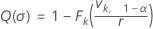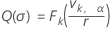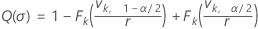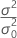# Methods and formulas for Power and Sample Size for 1 Variance

Select the method or formula of your choice.

## Calculating power

The power function for a variance test is Q(σ) = P(Reject H0 | σ)

### One-sided power (H1: σ > σ0)### One-sided power (H1: σ < σ0)### Two-sided power (H1: σ ≠ σ0)### Notation

TermDescription
Fk cumulative distribution function of chi-square distribution with k degrees of freedom
k n – 1
vk, A inverse CDF evaluated at A for a chi-square distribution with k degrees of freedom
α significance level
r## Calculating sample size and ratio

If you provide values for power and sample size, Minitab calculates the value of the ratio. If you provide values for power and ratio, Minitab calculates the value of the sample size.

For these two cases, Minitab uses an iterative algorithm with the power equation. At each iteration, Minitab evaluates the power for a trial sample size or trial ratio, and stops when it reaches the values you request.

### Target power and actual power

When Minitab calculates sample size, it may find that no integer value of sample size yields your target power. In such cases, Minitab displays the target value for power alongside the actual power, which is a value corresponding to an integer sample size, and which is nearest to, yet greater than, the target value.

By using this site you agree to the use of cookies for analytics and personalized content.  Read our policy